Courses

# Electric Current, Ohm's Law and Drift of Electrons Class 12 Notes | EduRev

## Class 12 : Electric Current, Ohm's Law and Drift of Electrons Class 12 Notes | EduRev

The document Electric Current, Ohm's Law and Drift of Electrons Class 12 Notes | EduRev is a part of the Class 12 Course Physics Class 12.
All you need of Class 12 at this link: Class 12

1. Introduction

• Conductor:
In some materials, the outer electrons of each atom or molecules are only weakly bound to it. These electrons are almost free to move throughout the body of the material and are called free electrons. They are also known as conduction electrons. When such a material is placed in an electric field, the free electrons move in a direction opposite to the field. Such materials are called conductors.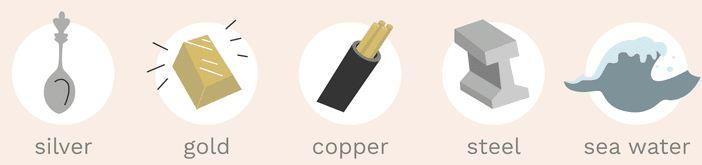Fig: Examples of conductors
• Insulator:
Another class of materials is called insulators in which all the electrons are tightly bound to their respective atoms or molecules. Effectively, there are no free electrons. When such a material is placed in an electric field, the electrons may slightly shift opposite to the field but they can't leave their parent atoms or molecules and hence can't move through long distances. Such materials are also called dielectrics.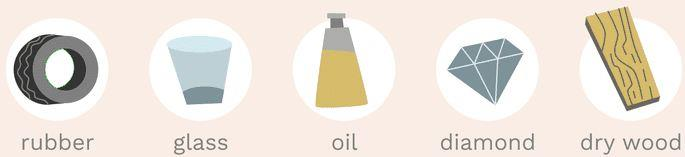Fig: Examples of insulators
• Semiconductor:
In semiconductors, the behavior is like an insulator at low levels of temperature. But at higher temperature, a small number of electrons are able to free themselves and they respond to the applied electric field. As the number of free electrons in a semiconductor is much smaller than that in a conductor, its behavior is in between a conductor and an insulator and hence, the name semiconductor. A freed electron in a semiconductor leaves a vacancy in its normal bound position. These vacancies also help in conduction.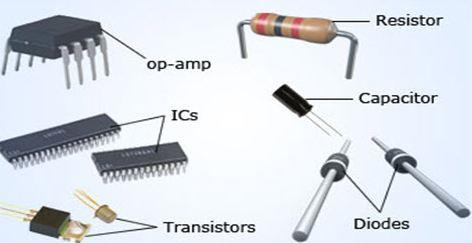Fig: Semiconductors2. Electric Current and Current Density:
When there is a transfer of charge from one side of an area to the other, we say that there is electric current through the area. If the moving charges are positive, the current is in the direction of motion, if they are negative, the current is opposite to the direction of motion. If a charge ΔQ crosses an area in time Δt, we define the average electric current through the area during this time as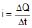The current at time t is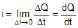Thus, electric current through an area is the rate of transfer of charge from one side of the area to the other. The SI unit of current is ampere. If one coulomb of charge crosses an area in one second, the current is one ampere. It is one of the seven base units accepted in SI.

Ex. 1 If q = 2t2  3, find current at t = 2 sec ?

Ans: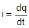i = 4t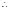i at 2 sec = 4 × 2 = 8 A

We shall now define a vector quantity known as electric current density at a point. To define the current density at a point P, we draw a small area DS through P perpendicular to the flow of charges(shown in figure) If Di be the current through the area DS, the average current density is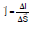The current density at the point P is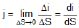The direction of the current density is the same as the direction of the current. Thus, it is along the motion of the moving charges, if the charges are positive and opposite to the motion of the charges, if the charges are negative. If a current i is uniformly distributed over an area S and is perpendicular to it,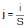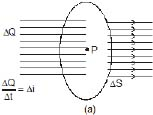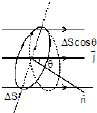Now let us consider an area DS which is not necessarily perpendicular to the current (figure shown) If the normal to the area makes an angle q with the direction of the current, the current density is,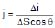or, Δi = j ΔS cos q

where Δi is the current through ΔS, If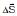be the area vector corresponding to the area ΔS, we have

For a finite area,

Note carefully that an electric current has direction as well as magnitude but it is not a vector quantity. It does not add like vectors. Therefore current is neither a vector quantity nor a scalar quantity but a tensor quantity. The current density is a vector quantity.

Ex. 2 An electron beam has an aperture 1.0 mm2. A total of 6.0 × 1010 electrons go through any perpendicular cross-section per second. Find (a) the current and (b) the current density in the beam.

Ans: The total charge crossing a perpendicular cross-section in one second is

q = ne

= 6.0 × 1016 × 1.6 × 10-19 C

= 9.6 × 10-3 C

The current is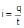=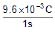= 9.6 × 10-3 A

As the charge is negative, the current is opposite to be direction of motion of the beam.

(b) The current density is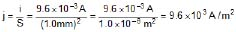3. Drift Speed

A conductor contains a large number of loosely bound electrons which we call free electrons or conduction electrons. The remaining material is a collection of relatively heavy positive ions which we call lattice. These ions keep on vibrating about their mean positions. The average amplitude depends on the temperature. Occasionally, a free electron collides or interacts in some other fashion with the lattice. The speed and direction of the electron changes randomly at each such event. As a result, the electron moves in a zig-zag path. As there are a large number of free electrons moving in random directions, the number of electrons crossing an area DS from one side very nearly equals the number crossing from the other side at any given time interval. The electric current through the area is, therefore, zero.

When there is an electric field inside the conductor, a force acts on each electron in the direction opposite to the field. The electrons get biased in their random motion in favor of the force. As a result, the electrons drift slowly in this direction. At each collision, the electron starts afresh in a random direction with a random speed but gains an additional velocity v' due to the electric field. This velocity v' increases with time and suddenly becomes zero as the electron makes a collision with the lattice and starts afresh with a random velocity. As the time, t between successive collisions is small, the electron "slowly and steadily drifts opposite to the applied field (shown figure). If the electron drifts a distance l in a long time t, we define drift speed as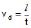If t be the average time between successive collisions, the distance drifted during this period is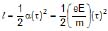The drift speed is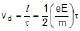It is proportional to the electric field E and to the average collision-time t.

The random motion of free electrons does not contribute to the drift of these electrons. Also, the average collision-time is constant for a given material at a given temperature. We, therefore, make the following assumption for our present purpose of discussing electric current.

When no electric field exists in a conductor, the free electrons stay at rest (Vd = 0) and when a field E exists, they move with a constant velocity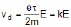...(1)

opposite to the field. The constant k depends on the material of the conductor and its temperature.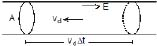Let us now find the relation between the current density and the drift speed. Consider a cylindrical conductor of cross-sectional area A in which an electric field E exists. Consider a length vd Δt of the conductor (figure shown). The volume of this portion is AvdΔt. If there are n free electrons per unit volume of the wire, the number of free electrons in this portion is nAvdΔt. All these electrons cross the area A in time Δt. Thus, the charge crossing this area in time Δt is

ΔQ = nAvd Δt e

or,= nAvde

and j =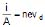...(2)

Ex. 3 Calculate the drift speed of the electrons when 1 A of current exists in a copper wire of cross-section 2 mm2. The number of free electrons in 1 cm3 of copper is 8.5 × 1022.

Ans: We have

j = nevd

or,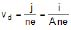=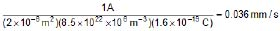We see that the drift speed is indeed small.

4. OHM'S LAW:

Using equations (1) and (2)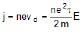or,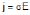...(3)

where s =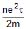where s depends only on material of the conductor and its temperature. This constant is called the electrical conductivity of the material. Equation (3) is known as Ohm's law.

The resistivity of a material is defined as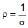=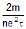...(4)

Ohm's law tells us that the conductivity (or resistivity) of a material is independent of the electric field existing in the material. This is valid for conductors over a wide range of field.

Suppose we have a conductor of length l and uniform cross-sectional area A (figure shown) Let us apply a potential difference V between the ends of the conductor. The electric field inside the conductor is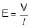. If the current in the conductor is i, the current density is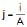. Ohm's law j = sE then becomes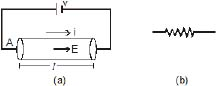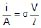or,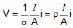...(5)

or, V = R i ...(6)

R is called the resistance of the given conductor. The quantity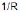is called conductance.

Equation (5) is another form of Ohm's law which is widely used in circuit analysis. The unit of resistance is called ohm and is denoted by symbol W. An object of conducting material, having a resistance of desired value, is called a resistor.

From equation (5) and (6)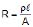...(7)

From equation (7), the unit of resistivity ρ is ohm-metre, also written as ?-m. The unit of conductivity (σ) is (ohm-m) written as ohm/m.

from eq. (4) & (7)

where r = resistivity

l = length along the direction of current

A = Area of the cross section perpendicular to direction of current

n = no. of free charges per unit volume.

τ = relaxation time

m = mass of electron

Ex. 4 Calculate the resistance of an aluminium wire of length 50 cm and cross-sectional area 2.0 mm2. The resistivity of aluminium is ρ = 2.6 × 10-8m ?

Ans: The resistance is R =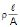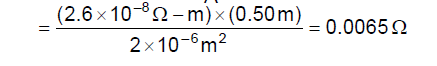We arrived at Ohm's law by making several assumptions about the existence and behaviour of the free electrons. These assumption are not valid for semiconductors, insulators, solutions etc. Ohm's law cannot be applied in such cases.

Ex. 5 The dimensions of a conductor of specific resistance r are shown below. Find the resistance of the conductor across AB, CD and EF.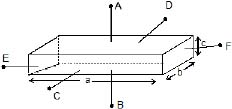Ans: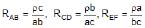Ex. 6 A portion of length L is cut out of a conical solid wire. The two ends of this portion have circular cross-sections of radii r1 and r2 (r2 > r1). It is connected lengthwise to a circuit and a current i is flowing in it. The resistivity of the material of the wire is ρ. Calculate the resistance of the considered portion and the voltage developed across it.

Ans: If follows from the figure, that

tan =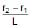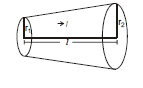Therefore, r = r1  x tan q = r1  x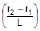=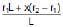Therefore,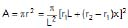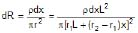⇒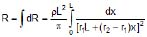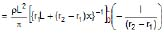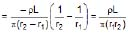Therefore,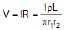Ex. 7 The space between two coaxial cylinders, whose radii are a and b (where a < b as in (figure shown) is filled with a conducting medium. The specific conductivity of the medium is σ.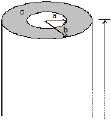(a) Compute the resistance along the length of cylinder.

(b) Compute the resistance between the cylinders in the radial direction. Assume that the cylinders are very long as compared to their radii, i.e., L >> b, where L is the length of the cylinders.

Ans: (a) R =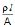=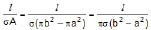(b) From Ohm's law, we have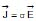Assuming radial current density.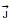becomes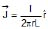for a < r < b

and, therefore,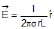Here we have used the assumption that L >> b so that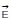and are in cylindrically symmetric form. The potential drop across the medium is thus :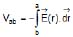=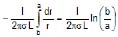The resistance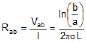Method 2: We split the medium into differential cylindrical shell elements of width dr, in series. The current flow is cylindrically symmetric (L >> b). The area through which the current flows across a shell of radius r is A(r) = 2πrL. The length the current flows, passing through a shell of radius r is dr. Therefore, the resistance of the shell of radius r is :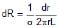Since the shells are connected in a series, we have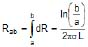Offer running on EduRev: Apply code STAYHOME200 to get INR 200 off on our premium plan EduRev Infinity!

,

,

,

,

,

,

,

,

,

,

,

,

,

,

,

,

,

,

,

,

,

,

,

,

;